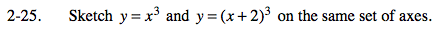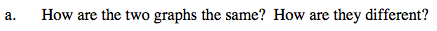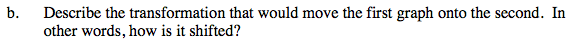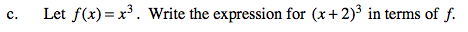### Home > PC > Chapter 2 > Lesson 2.1.2 > Problem2-25

2-25.
1. Sketch y = x3 and y = (x + 2)3 on the same set of axes. 2-25 HW eTool (Desmos) Homework Help ✎

1. How are the two graphs the same? How are they different?

2. Describe the transformation that would move the first graph onto the second. In other words, how is it shifted?

3. Let f(x) = x3. Write the expression for (x + 2)3 in terms of f.The second graph is the first graph shifted to the left 2 units.f(x + 2) = (x + 2)3

Use the eTool below to view the graphs and to graph f(x) when it is transformed to match y.
Click the link at right for the full version of the eTool: PCT 2-25 HW eTool